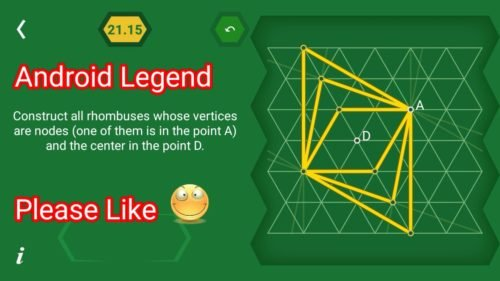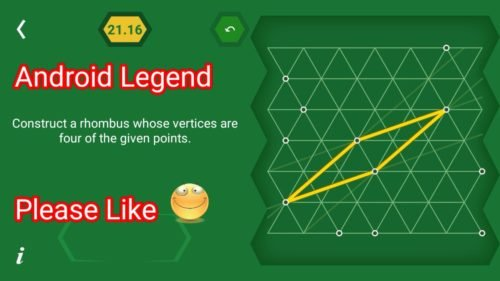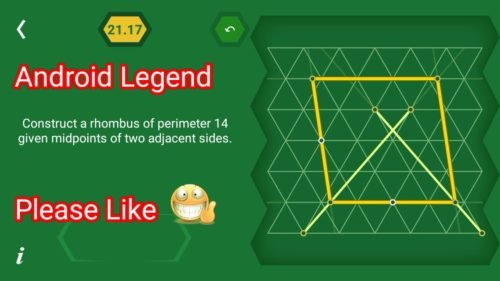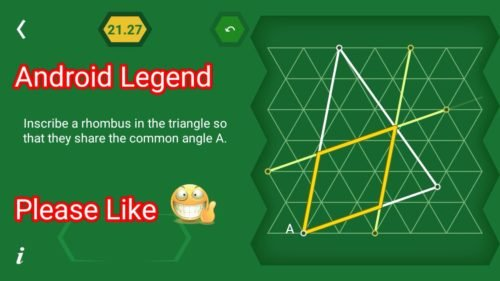# Pythagorea 60 degree Level 21.1 21.2 21.3 21.4 21.5 21.6 21.7 21.8 21.9 21.10 21.11 21.12 21.13 21.14 21.15 21.16 21.17 21.18 21.19 21.20 21.21 21.22 21.23 21.24 21.25 21.26 21.27 Solutions/AnswersPythagorea 60 degrees is android/iOS app developed by Horis International Limited. This game is mostly focused on geometric puzzles and construction. The workspace is divided into triangular grids lines aligned 60° to each other, that’s why this game names as pythagorea 60°. You should know all the basic Math operations. All lines and shapes are drawn on a grid whose cells are equilateral triangles. Most of the game levels can be answered using natural intuition and bye some basic laws of geometry.

You have to connect points on the grid using straight lines to construct an element, you can even use intersection points to draw. some levels are very easy some are of medium difficulty and some are very hard to solve, To help you, I am providing solutions to all the problems.

If you are here for levels other than ‘Rhombuses’ Go to directory of all other levels at : http://www.puzzlegamemaster.com/pythagorea-60-complete/

• Pythagorea 60° Level 21.1: Connect the four given points to get a rhombus.• Pythagorea 60° Level 21.2: Construct a rhombus given three of its Vertices.• Pythagorea 60° Level 21.3: Construct all rhombuses whose Vertices are nodes given two of them.• Pythagorea 60° Level 21.4: Construct all rhombuses whose Vertices are nodes given two of them.• Pythagorea 60° Level 21.5: Construct all rhombuses with angle 60 given two opposite vertices.• Pythagorea 60° Level 21.6: Construct a rhombus whose Vertices are four of the given points.• Pythagorea 60° Level 21.7: Construct all rhombuses whose vertices are nodes (two of them are given) and whose diagonal is of length 6.• Pythagorea 60° Level 21.8: Construct all rhombuses whose vertices are nodes (two of them are given) and whose diagonal is of length 3√7.• Pythagorea 60° Level 21.9: Construct all rhombuses whose Vertices are nodes (one of them is in the point A) and with one diagonal of length 1 and the other of length 3√3.• Pythagorea 60° Level 21.10: Construct all rhombuses whose Vertices are nodes (one of them is in the point A) and Whose diagonal is of length 4.• Pythagorea 60° Level 21.11: Construct all rhombuses whose Vertices are nodes (one of them is in the point A) and Whose diagonal is of length 5.• Pythagorea 60° Level 21.12: Construct all rhombuses whose Vertices are nodes (one of them is in the point A) and the midpoint of a side in the point S.• Pythagorea 60° Level 21.13: Construct all rhombuses whose Vertices are nodes given the midpoints of two adjacent sides.• Pythagorea 60° Level 21.14: Construct all rhombuses with a vertex in the point A given midpoints of two sides.• Pythagorea 60° Level 21.15: Construct all rhombuses whose Vertices are nodes (one of them is in the point A) and the center in the point D.• Pythagorea 60° Level 21.16: Construct a rhombus whose Vertices are four of the given points.• Pythagorea 60° Level 21.17: Construct a rhombus of perimeter 14 given midpoints of two adjacent sides.• Pythagorea 60° Level 21.18: Construct a rhombus whose Vertices are four of the given points.• Pythagorea 60° Level 21.19: Inscribe a rhombus in the rectangle SO that they share a diagonal.• Pythagorea 60° Level 21.20: Inscribe a rhombus in the rectangle SO that they share a diagonal.• Pythagorea 60° Level 21.21: Inscribe a rhombus in a given rhombus so that they have the same center. One of its vertices is in the point A.• Pythagorea 60° Level 21.22: Inscribe a rhombus in a given rhombus so that they have the same center. One of its vertices is in the point A.• Pythagorea 60° Level 21.23: Inscribe a rhombus in the triangle so that they share the Common angle A.• Pythagorea 60° Level 21.24: Inscribe a rhombus in the triangle so that they share the Common angle A.• Pythagorea 60° Level 21.25: Inscribe a rhombus in the triangle so that they share the Common angle A.• Pythagorea 60° Level 21.26: Inscribe a rhombus in the triangle so that they share the Common angle A.• Pythagorea 60° Level 21.27: Inscribe a rhombus in the triangle so that they share the Common angle A.Feel free to comment below if you have any doubts regarding the solutions. I will try to help you guys. All other levels are posted on this blog please visit them too.